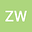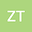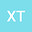Global existence and decay estimate of solution to compressible quantum Navier-Stokes equations with damping
•••• Zhonger Wu,
• Zhong Tan,
• Xu TANG
Zhonger Wu
Xiamen University

Corresponding Author:wze622520@163.com

Author ProfileZhong Tan
Xiamen University
Author ProfileXu TANG
Sichuan University
Author Profile## Abstract

In this paper, we consider the Cauchy problem of the compressible quantum Navier-Stokes equations with damping in R3. We first assume that the H3-norm of the initial data is sufficiently small while the higher derivative can be arbitrarily large, and prove the global existence of smooth solutions. Then the decay estimate of the solution is derived for the initial data in a homogeneous Sobolev space or Besov space with negative exponent. In addition, the usual Lp−L2(1 ≤ p ≤ 2) type decay rate is obtained without assuming that the Lpnorm of the initial data is sufficiently small.